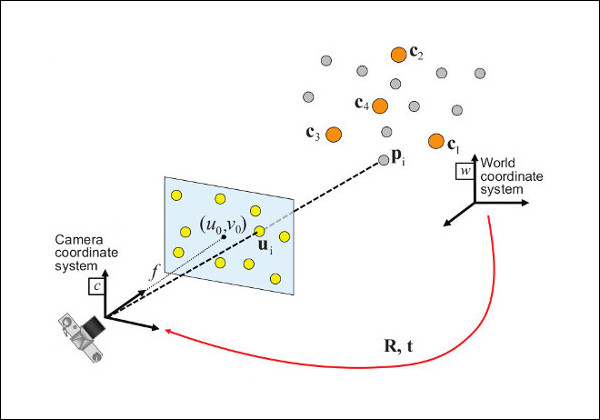OpenCV  3.4.20-dev Open Source Computer Vision
Perspective-n-Point (PnP) pose computation

## Pose computation overview

The pose computation problem  consists in solving for the rotation and translation that minimizes the reprojection error from 3D-2D point correspondences.

The solvePnP and related functions estimate the object pose given a set of object points, their corresponding image projections, as well as the camera intrinsic matrix and the distortion coefficients, see the figure below (more precisely, the X-axis of the camera frame is pointing to the right, the Y-axis downward and the Z-axis forward).Points expressed in the world frame $$\bf{X}_w$$ are projected into the image plane $$\left[ u, v \right]$$ using the perspective projection model $$\Pi$$ and the camera intrinsic parameters matrix $$\bf{A}$$ (also denoted $$\bf{K}$$ in the literature):

\begin{align*} \begin{bmatrix} u \\ v \\ 1 \end{bmatrix} &= \bf{A} \hspace{0.1em} \Pi \hspace{0.2em} ^{c}\bf{T}_w \begin{bmatrix} X_{w} \\ Y_{w} \\ Z_{w} \\ 1 \end{bmatrix} \\ \begin{bmatrix} u \\ v \\ 1 \end{bmatrix} &= \begin{bmatrix} f_x & 0 & c_x \\ 0 & f_y & c_y \\ 0 & 0 & 1 \end{bmatrix} \begin{bmatrix} 1 & 0 & 0 & 0 \\ 0 & 1 & 0 & 0 \\ 0 & 0 & 1 & 0 \end{bmatrix} \begin{bmatrix} r_{11} & r_{12} & r_{13} & t_x \\ r_{21} & r_{22} & r_{23} & t_y \\ r_{31} & r_{32} & r_{33} & t_z \\ 0 & 0 & 0 & 1 \end{bmatrix} \begin{bmatrix} X_{w} \\ Y_{w} \\ Z_{w} \\ 1 \end{bmatrix} \end{align*}

The estimated pose is thus the rotation (rvec) and the translation (tvec) vectors that allow transforming a 3D point expressed in the world frame into the camera frame:

\begin{align*} \begin{bmatrix} X_c \\ Y_c \\ Z_c \\ 1 \end{bmatrix} &= \hspace{0.2em} ^{c}\bf{T}_w \begin{bmatrix} X_{w} \\ Y_{w} \\ Z_{w} \\ 1 \end{bmatrix} \\ \begin{bmatrix} X_c \\ Y_c \\ Z_c \\ 1 \end{bmatrix} &= \begin{bmatrix} r_{11} & r_{12} & r_{13} & t_x \\ r_{21} & r_{22} & r_{23} & t_y \\ r_{31} & r_{32} & r_{33} & t_z \\ 0 & 0 & 0 & 1 \end{bmatrix} \begin{bmatrix} X_{w} \\ Y_{w} \\ Z_{w} \\ 1 \end{bmatrix} \end{align*}

## Pose computation methods

Refer to the cv::SolvePnPMethod enum documentation for the list of possible values. Some details about each method are described below:

• cv::SOLVEPNP_ITERATIVE Iterative method is based on a Levenberg-Marquardt optimization. In this case the function finds such a pose that minimizes reprojection error, that is the sum of squared distances between the observed projections "imagePoints" and the projected (using cv::projectPoints ) "objectPoints". Initial solution for non-planar "objectPoints" needs at least 6 points and uses the DLT algorithm. Initial solution for planar "objectPoints" needs at least 4 points and uses pose from homography decomposition.
• cv::SOLVEPNP_P3P Method is based on the paper of X.S. Gao, X.-R. Hou, J. Tang, H.-F. Chang "Complete Solution Classification for the Perspective-Three-Point Problem" (). In this case the function requires exactly four object and image points.
• cv::SOLVEPNP_AP3P Method is based on the paper of T. Ke, S. Roumeliotis "An Efficient Algebraic Solution to the Perspective-Three-Point Problem" (). In this case the function requires exactly four object and image points.
• cv::SOLVEPNP_EPNP Method has been introduced by F. Moreno-Noguer, V. Lepetit and P. Fua in the paper "EPnP: Efficient Perspective-n-Point Camera Pose Estimation" ().
• cv::SOLVEPNP_DLS Broken implementation. Using this flag will fallback to EPnP.
Method is based on the paper of J. Hesch and S. Roumeliotis. "A Direct Least-Squares (DLS) Method for PnP" ().
• cv::SOLVEPNP_UPNP Broken implementation. Using this flag will fallback to EPnP.
Method is based on the paper of A. Penate-Sanchez, J. Andrade-Cetto, F. Moreno-Noguer. "Exhaustive Linearization for Robust Camera Pose and Focal Length Estimation" (). In this case the function also estimates the parameters $$f_x$$ and $$f_y$$ assuming that both have the same value. Then the cameraMatrix is updated with the estimated focal length.
• cv::SOLVEPNP_IPPE Method is based on the paper of T. Collins and A. Bartoli. "Infinitesimal Plane-Based Pose Estimation" (). This method requires coplanar object points.
• cv::SOLVEPNP_IPPE_SQUARE Method is based on the paper of Toby Collins and Adrien Bartoli. "Infinitesimal Plane-Based Pose Estimation" (). This method is suitable for marker pose estimation. It requires 4 coplanar object points defined in the following order:
• point 0: [-squareLength / 2, squareLength / 2, 0]
• point 1: [ squareLength / 2, squareLength / 2, 0]
• point 2: [ squareLength / 2, -squareLength / 2, 0]
• point 3: [-squareLength / 2, -squareLength / 2, 0]
• cv::SOLVEPNP_SQPNP Method is based on the paper "A Consistently Fast and Globally Optimal Solution to the Perspective-n-Point Problem" by G. Terzakis and M.Lourakis (). It requires 3 or more points.

## P3P

The cv::solveP3P() computes an object pose from exactly 3 3D-2D point correspondences. A P3P problem has up to 4 solutions.

Note
The solutions are sorted by reprojection errors (lowest to highest).

## PnP

The cv::solvePnP() returns the rotation and the translation vectors that transform a 3D point expressed in the object coordinate frame to the camera coordinate frame, using different methods:

• P3P methods (cv::SOLVEPNP_P3P, cv::SOLVEPNP_AP3P): need 4 input points to return a unique solution.
• cv::SOLVEPNP_IPPE Input points must be >= 4 and object points must be coplanar.
• cv::SOLVEPNP_IPPE_SQUARE Special case suitable for marker pose estimation. Number of input points must be 4. Object points must be defined in the following order:
• point 0: [-squareLength / 2, squareLength / 2, 0]
• point 1: [ squareLength / 2, squareLength / 2, 0]
• point 2: [ squareLength / 2, -squareLength / 2, 0]
• point 3: [-squareLength / 2, -squareLength / 2, 0]
• for all the other flags, number of input points must be >= 4 and object points can be in any configuration.

## Generic PnP

The cv::solvePnPGeneric() allows retrieving all the possible solutions.

Currently, only cv::SOLVEPNP_P3P, cv::SOLVEPNP_AP3P, cv::SOLVEPNP_IPPE, cv::SOLVEPNP_IPPE_SQUARE, cv::SOLVEPNP_SQPNP can return multiple solutions.

## RANSAC PnP

The cv::solvePnPRansac() computes the object pose wrt. the camera frame using a RANSAC scheme to deal with outliers.